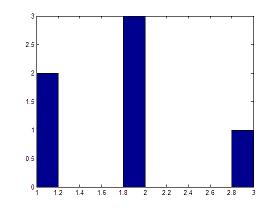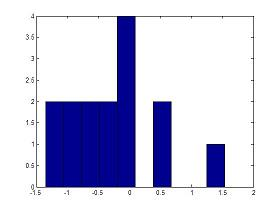# hist - Histograms in Matlab

### Commands for histograms and vertical data

The hist instruction in Matlab, without output arguments, produces a histogram bar plot of the results.

The bar edges on the first and last bins may extend to cover the min and max of the data unless a matrix of data is supplied.

A histogram is a graphical representation used to estimate the probability distribution of a variable. It counts the frequencies  of data into discrete intervals (bins), and then plots a rectangle over each interval.

Example 1:

This Matlab code creates a histogram with 3 bars. The first bar has two '1' values, the second bar has three '2' values, and the third bar has one '3' values.

y = [1 1 2 2 2 3]
hist(y)The horizontal axis has the different values in the vector to be plotted.
The vertical axis has the number of those values in the vector.

Example 2:

This Matlab code generates a histogram of 15 randomly distributed numbers between 0 and 1 (you can type 'help rand' on your command window to learn about random numbers).

a = randn(15,1)
hist(a)

a =
-0.8468
-0.2463
0.6630
-0.8542
-1.2013
-0.1199
-0.0653
0.4853
-0.5955
-0.1497
-0.4348
-0.0793
1.5352
-0.6065
-1.3474

>>Note that each column includes a range of values, otherwise the histogram would contain 15 bars.

You can type ' help hist ' or ' help bar ' on your command window to see more details of use.